xModified Newtonian dynamicsEncyclopedia
In physics
Physics
Physics is a natural science that involves the study of matter and its motion through spacetime, along with related concepts such as energy and force. More broadly, it is the general analysis of nature, conducted in order to understand how the universe behaves.Physics is one of the oldest academic...

, Modified Newtonian dynamics (MOND) is a hypothesis that proposes a modification of Newton's law of gravity to explain the galaxy rotation problem
Galaxy rotation curve
The rotation curve of a galaxy can be represented by a graph that plots the orbital velocity of the stars or gas in the galaxy on the y-axis against the distance from the center of the galaxy on the x-axis....

. When the uniform velocity of rotation of galaxies was first observed, it was unexpected because Newtonian theory of gravity predicts that objects that are farther out will have lower velocities. For example, planet
Planet
A planet is a celestial body orbiting a star or stellar remnant that is massive enough to be rounded by its own gravity, is not massive enough to cause thermonuclear fusion, and has cleared its neighbouring region of planetesimals.The term planet is ancient, with ties to history, science,...

s in the Solar System
Solar System
The Solar System consists of the Sun and the astronomical objects gravitationally bound in orbit around it, all of which formed from the collapse of a giant molecular cloud approximately 4.6 billion years ago. The vast majority of the system's mass is in the Sun...

orbit with velocities that decrease as their distance from the Sun
Sun
The Sun is the star at the center of the Solar System. It is almost perfectly spherical and consists of hot plasma interwoven with magnetic fields...

increases.

MOND was proposed by Mordehai Milgrom
Mordehai Milgrom
Mordehai Milgrom is an Israeli physicist and professor in the department of Condensed Matter Physics at the Weizmann Institute in Rehovot, Israel. He is most famous for his proposal of Modified Newtonian dynamics as an alternative to the dark matter and galaxy rotation curve problems, in 1981...

in 1983 as a way to model this observed uniform velocity data. Milgrom noted that Newton's law for gravitational force has been verified only where gravitational acceleration is large, and suggested that for extremely low accelerations the theory may not hold. MOND theory posits that acceleration
Acceleration
In physics, acceleration is the rate of change of velocity with time. In one dimension, acceleration is the rate at which something speeds up or slows down. However, since velocity is a vector, acceleration describes the rate of change of both the magnitude and the direction of velocity. ...

is not linearly proportional to force
Force
In physics, a force is any influence that causes an object to undergo a change in speed, a change in direction, or a change in shape. In other words, a force is that which can cause an object with mass to change its velocity , i.e., to accelerate, or which can cause a flexible object to deform...

at low values.

MOND stands in contrast to the more widely accepted theory of dark matter
Dark matter
In astronomy and cosmology, dark matter is matter that neither emits nor scatters light or other electromagnetic radiation, and so cannot be directly detected via optical or radio astronomy...

. Dark matter theory suggests that each galaxy contains a halo
Dark matter halo
A dark matter halo is a hypothetical component of a galaxy, which extends beyond the edge of the visible galaxy and dominates the total mass. Since they consist of dark matter, halos cannot be observed directly, but their existence is inferred through their effects on the motions of stars and gas...

of an as yet unidentified type of matter that provides an overall mass distribution different from the observed distribution of normal matter
Matter
Matter is a general term for the substance of which all physical objects consist. Typically, matter includes atoms and other particles which have mass. A common way of defining matter is as anything that has mass and occupies volume...

. This dark matter accounts for the uniform rotation velocity data without modifying Newton's law of gravity.

## Overview: Galaxy dynamics

Observations of the rotation rates of spiral galaxies
Galaxy
A galaxy is a massive, gravitationally bound system that consists of stars and stellar remnants, an interstellar medium of gas and dust, and an important but poorly understood component tentatively dubbed dark matter. The word galaxy is derived from the Greek galaxias , literally "milky", a...

began in 1978. By the early 1980s it was clear that galaxies did not exhibit the same pattern of decreasing orbital velocity with increasing distance from the center of mass observed in the Solar System. A spiral galaxy
Spiral galaxy
A spiral galaxy is a certain kind of galaxy originally described by Edwin Hubble in his 1936 work The Realm of the Nebulae and, as such, forms part of the Hubble sequence. Spiral galaxies consist of a flat, rotating disk containing stars, gas and dust, and a central concentration of stars known as...

consists of a bulge
Bulge (astronomy)
In astronomy, a bulge is a tightly packed group of stars within a larger formation. The term almost exclusively refers to the central group of stars found in most spiral galaxies...

of star
Star
A star is a massive, luminous sphere of plasma held together by gravity. At the end of its lifetime, a star can also contain a proportion of degenerate matter. The nearest star to Earth is the Sun, which is the source of most of the energy on Earth...

s at the centre with a vast disc of stars orbiting around the central group. If the orbits of the stars were governed solely by gravitational force and the observed distribution of normal matter, it was expected that stars at the outer edge of the disc would have a much lower orbital velocity than those near the middle. In the observed galaxies this pattern is not apparent. Stars near the outer edge orbit the centre of the galaxy at the same speed as stars closer to the middle.The dotted curve A in Figure 1 at left shows the predicted orbital velocity as a function of distance from the galactic center assuming neither MOND nor dark matter. The solid curve B shows the observed distribution. Instead of decreasing asymptotically to zero as the effect of gravity wanes, this curve remains flat, showing the same velocity at increasing distances from the bulge. Astronomers call this phenomenon the "flattening of galaxies' rotation curves".

Scientists hypothesized that the flatness of the rotation of galaxies is caused by matter outside the galaxy's visible disc. Since all large galaxies show the same characteristic, large galaxies must, according to this line of reasoning, be embedded in a halo of invisible "dark" matter
Dark matter
In astronomy and cosmology, dark matter is matter that neither emits nor scatters light or other electromagnetic radiation, and so cannot be directly detected via optical or radio astronomy...

.

## The MOND Theory

In 1983, Mordehai Milgrom
Mordehai Milgrom
Mordehai Milgrom is an Israeli physicist and professor in the department of Condensed Matter Physics at the Weizmann Institute in Rehovot, Israel. He is most famous for his proposal of Modified Newtonian dynamics as an alternative to the dark matter and galaxy rotation curve problems, in 1981...

, a physicist at the Weizmann Institute in Israel, published three papers in Astrophysical Journal
Astrophysical Journal
The Astrophysical Journal is a peer-reviewed scientific journal covering astronomy and astrophysics. It was founded in 1895 by the American astronomers George Ellery Hale and James Edward Keeler. It publishes three 500-page issues per month....

to propose a modification of Newton's law of gravity. A pedagogical introduction to MOND can be found in Bekenstein, who characterizes MOND as follows: "Relativistic MOND as here described has developed from the ground up, rather than coming down from the sky: phenomenology
Phenomenology (science)
The term phenomenology in science is used to describe a body of knowledge that relates empirical observations of phenomena to each other, in a way that is consistent with fundamental theory, but is not directly derived from theory. For example, we find the following definition in the Concise...

, rather than pure theoretical ideas."

Actually, Milgrom provided several interpretations of his proposal, one being a modification of Newton's second law of motion. However, this proposed interpretation is inconsistent with conservation of momentum, requiring some unconventional physical assumptions to regain plausibility. A second interpretation, as a modification of the law of gravity, requires that the acceleration due to gravitational force does not depend simply upon the mass m, but upon the form m/μ(a/a0), where μ is some function approaching the value one for large arguments and a/a0 for small arguments, and a is the acceleration caused by gravity and a0 is a natural constant, a0 ≈ 10−10 m/s2. The centripetal accelerations of stars and gas clouds at the outskirts of spiral galaxies tend to be below a0.

The exact form of µ is unspecified, only its behavior when the argument a/a0 is small or large. As Milgrom proved in his original paper, the form of µ does not change most of the consequences of the theory, such as the flattening of the rotation curve.

In the everyday world, a is much greater than a0 for all physical effects, therefore µ(a/a0)=1 and F=ma as usual. Consequently, the change in Newton's law of gravity is negligible and Newton could not have seen it.

### Predicted rotation curve

Far away from the center of a galaxy, the gravitational force a star undergoes is, with good approximation: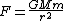with G the gravitation constant
Physical constant
A physical constant is a physical quantity that is generally believed to be both universal in nature and constant in time. It can be contrasted with a mathematical constant, which is a fixed numerical value but does not directly involve any physical measurement.There are many physical constants in...

, M the mass of the galaxy, m the mass of the star and r the distance between the center and the star. Using the new law of dynamics gives: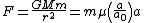Eliminating m gives: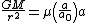Assuming that, at this large distance r, a is smaller than a0,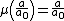. This gives: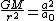Therefore: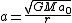Since the equation that relates the velocity to the acceleration for a circular orbit
Orbit
In physics, an orbit is the gravitationally curved path of an object around a point in space, for example the orbit of a planet around the center of a star system, such as the Solar System...

is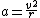, one has: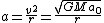and therefore: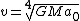Consequently, the velocity of stars on a circular orbit far from the center is a constant, and does not depend on the distance r : the rotation curve is flat.

The proportion between the "flat" rotation velocity to the observed mass derived here is matching the observed relation between "flat" velocity to luminosity known as the Tully-Fisher relation
Tully-Fisher relation
In astronomy, the Tully–Fisher relation, published by astronomers R. Brent Tully and J. Richard Fisher in 1977, is an empirical relationship between the intrinsic luminosity of a spiral galaxy and its velocity width...

.

At the same time, there is a clear relationship between the velocity and the constant a0. The equation v=(GMa0)1/4 allows one to calculate a0 from the observed v and M. Milgrom found a0=1.2×10−10 ms−2.

To explain the meaning of this constant, Milgrom said : "... It is roughly the acceleration that will take an object from rest to the speed of light in the lifetime of the universe. It is also of the order of the recently discovered acceleration of the universe."

Retrospectively, the impact of assumed value of a>>a0 for physical effects on Earth remains valid. Had a0 been larger, its consequences would have been visible on Earth and, since it is not the case, the new theory would have been inconsistent.

## Consistency with the observations

According to the Modified Newtonian Dynamics theory, every physical process that involves small accelerations due to gravity will have an outcome different from that predicted by the simple law F=ma. Therefore, astronomers need to look for all such processes and verify that MOND remains compatible with observations, that is, within the limit of the uncertainties on the data. There is, however, a complication overlooked up to this point but that strongly affects the compatibility between MOND and the observed world: in a system considered as isolated, for example a single satellite
Satellite
In the context of spaceflight, a satellite is an object which has been placed into orbit by human endeavour. Such objects are sometimes called artificial satellites to distinguish them from natural satellites such as the Moon....

orbiting a planet
Planet
A planet is a celestial body orbiting a star or stellar remnant that is massive enough to be rounded by its own gravity, is not massive enough to cause thermonuclear fusion, and has cleared its neighbouring region of planetesimals.The term planet is ancient, with ties to history, science,...

, the effect of MOND results in an increased velocity beyond a given range (actually, below a given acceleration, but for circular orbits it is the same thing) that depends on the mass of both the planet and the satellite. However, if the same system is actually orbiting a star, the planet and the satellite will be accelerated in the star's gravitational field. For the satellite, the sum of the two fields could yield acceleration greater than a0, and the orbit would not be the same as that in an isolated system.

For this reason, the typical acceleration of any physical process is not the only parameter astronomers must consider. Also critical is the process's environment, which is all external forces that are usually neglected. In his paper, Milgrom arranged the typical acceleration of various physical processes in a two-dimensional diagram. One parameter is the acceleration of the process itself, the other parameter is the acceleration induced by the environment.

This affects MOND's application to experimental observation and empirical data because all experiments done on Earth or its neighborhood are subject to the Sun's gravitational field, and this field is so strong that all objects in the Solar system undergo an acceleration greater than a0. This explains why the flattening of galaxies' rotation curve, or the MOND effect, had not been detected until the early 1980s, when astronomers first gathered empirical data on the rotation of galaxies.

Therefore, only galaxies and other large systems are expected to exhibit the dynamics that will allow astronomers to verify that MOND agrees with observation. Since Milgrom's theory first appeared in 1983, the most accurate data has come from observations of distant galaxies and neighbors of the Milky Way
Milky Way
The Milky Way is the galaxy that contains the Solar System. This name derives from its appearance as a dim un-resolved "milky" glowing band arching across the night sky...

. Within the uncertainties of the data, MOND has remained valid. The Milky Way itself is scattered with clouds of gas
Gas
Gas is one of the three classical states of matter . Near absolute zero, a substance exists as a solid. As heat is added to this substance it melts into a liquid at its melting point , boils into a gas at its boiling point, and if heated high enough would enter a plasma state in which the electrons...

and interstellar dust, and until now it has not been possible to draw a rotation curve for the galaxy. Finally, the uncertainties on the velocity of galaxies within clusters and larger systems have been too large to conclude in favor of or against MOND. Indeed, conditions for conducting an experiment that could confirm or disprove MOND may only be possible outside the Solar system. A couple of near-to-Earth tests of MOND have been proposed though: one involves flying the LISA Pathfinder
LISA Pathfinder
LISA Pathfinder is the revised name for SMART-2, a NASA/ESA space probe to be launched in June 2013. SMART stands for Small Missions for Advanced Research in Technology. The aim of the LISA Pathfinder is to test technologies needed for the Laser Interferometer Space Antenna, a joint NASA/ESA...

spacecraft through the Earth-Sun saddlepoint; another involves using a precisely controlled spinning disk to cancel out the acceleration effects of Earth's orbit around the Sun, and Sun's orbit around the galaxy; if either of these tests are carried out, and if MOND holds true, then they should feel a slight kick as they approach the very low acceleration levels required by MOND.

In search of observations that would validate his theory, Milgrom noticed that a special class of objects, the low surface brightness galaxies (LSB), is of particular interest: the radius of an LSB is large compared to its mass, and thus almost all stars are within the flat part of the rotation curve. Also, other theories predict that the velocity at the edge depends on the average surface brightness in addition to the LSB mass. Finally, no data on the rotation curve of these galaxies was available at the time. Milgrom thus could make the prediction that LSBs would have a rotation curve which is essentially flat, and with a relation between the flat velocity and the mass of the LSB identical to that of brighter galaxies.

Since then, the majority of LSBs observed has been consistent with the rotational curve predicted by MOND.

An exception to MOND other than LSB is prediction of the speeds of galaxies that gyrate around the center of a galaxy cluster. Our galaxy is part of the Virgo supercluster
Virgo Supercluster
The Virgo Supercluster or Local Supercluster is the irregular supercluster that contains the Virgo Cluster in addition to the Local Group, which in turn contains the Milky Way and Andromeda galaxies. At least 100 galaxy groups and clusters are located within its diameter of 33 megaparsecs...

. MOND predicts a rate of rotation of these galaxies about their center, and temperature distributions, that are contrary to observation.

Computer simulations show that MOND is generally very precise at predicting individual galaxy rotation curves, of all kinds of galaxies: spirals, ellipticals, dwarfs, etc. However, MOND and MOND-like theories are not so good at predicting galactic cluster-scale, or cosmological scale structures.

A test that might disprove MOND would be to discover any of the theorized Dark Matter particles, such as the WIMPs.

A recent proposal is that MOND successfully predicts the local galactic escape speed of the Milky Way, a measure of the mass beyond the galactocentric radius of the Sun.

Lee Smolin
Lee Smolin
Lee Smolin is an American theoretical physicist, a researcher at the Perimeter Institute for Theoretical Physics, and an adjunct professor of physics at the University of Waterloo. He is married to Dina Graser, a communications lawyer in Toronto. His brother is David M...

and co-workers have tried unsuccessfully to obtain a theoretical basis for MOND from quantum gravity
Quantum gravity
Quantum gravity is the field of theoretical physics which attempts to develop scientific models that unify quantum mechanics with general relativity...

. His conclusion is "MOND is a tantalizing mystery, but not one that can be resolved now."

In 2011 University of Maryland
University of Maryland
When the term "University of Maryland" is used without any qualification, it generally refers to the University of Maryland, College Park.University of Maryland may refer to the following:...

Astronomy Professor, Stacy McGaugh
Stacy McGaugh
Stacy McGaugh is an American astronomer and professor in the Department of Astronomy at the University of Maryland in College Park, Maryland. His fields of speciality include low surface brightness galaxies, galaxy formation and evolution, tests of dark matter and alternative hypotheses, and...

, examined the rotation of gas rich galaxies, which have relatively fewer stars and a prevalence of mass in the form of interstellar gas. This allowed the mass of the galaxy to be more accurately determined since matter in the form of gas is easier to see and measure than matter in the form of stars or planets. McGaugh studied a sample of 47 galaxies and compared each one's mass and speed of rotation with the ratio expected from MOND predictions. All 47 galaxies fell on or very close to the MOND prediction. No dark matter model performed as well. On the other hand, another 2011 study observing the gravity-induced redshift
Redshift
In physics , redshift happens when light seen coming from an object is proportionally increased in wavelength, or shifted to the red end of the spectrum...

of galactic clusters
Galaxy cluster
A galaxy cluster is a compact cluster of galaxies. Basic difference between a galaxy group and a galaxy cluster is that there are many more galaxies in a cluster than in a group. Also, galaxies in a cluster are more compact and have higher velocity dispersion. One of the key features of cluster is...

found results that strongly supported general relativity, but were inconsistent with MOND.

## The mathematics of MOND

In non-relativistic Modified Newtonian Dynamics, Poisson's equation
Poisson's equation
In mathematics, Poisson's equation is a partial differential equation of elliptic type with broad utility in electrostatics, mechanical engineering and theoretical physics...

,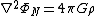(where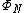is the gravitational potential and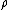is the density
distribution) is modified as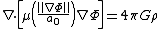where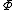is the MOND potential. The equation is to be solved
with boundary condition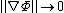for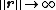.
The exact form of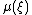is not constrained by observations, but must have
the behaviour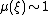for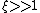(Newtonian regime),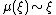for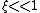(Deep-MOND regime).
In the deep-MOND regime, the modified Poisson equation may be rewritten as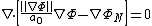and that simplifies to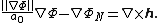The vector field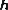is unknown, but is null whenever the density
distribution is spherical, cylindrical or planar. In that case, MOND acceleration field is
given by the simple formula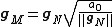where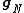is the normal Newtonian field.

## The External Field Effect (EFE)

In MOND it turns out that if a weakly gravitationally bound system s, whose inner accelerations are expected to be of the order of 10−10 m s−2 from a Newtonian calculation, is embedded in an external gravitational field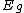generated by a larger array of masses S, then, even ifis uniform throughout the spatial extension of s, the internal dynamics of the latter is influenced byin such a way that the total acceleration within s is, actually, larger than 10−10 m s−2. In other words, the Strong Equivalence Principle
Equivalence principle
In the physics of general relativity, the equivalence principle is any of several related concepts dealing with the equivalence of gravitational and inertial mass, and to Albert Einstein's assertion that the gravitational "force" as experienced locally while standing on a massive body is actually...

is violated. Milgrom
Milgrom
Milgrom is a surname and may refer to:* Allen "Al" Milgrom, American comic book writer* Edwin Milgrom* Felix Milgrom, Jewish Polish-American microbiologist* Jacob Milgrom, Jewish Biblical scholar...

originally introduced such a concept to explain the fact that the expected phenomenology of dark matter
Dark matter
In astronomy and cosmology, dark matter is matter that neither emits nor scatters light or other electromagnetic radiation, and so cannot be directly detected via optical or radio astronomy...

—-to be explained in terms of MOND—-was absent just in some systems in which it should have, instead, been present. Those systems were some open globular clusters in the neighborhood of the Sun
Sun
The Sun is the star at the center of the Solar System. It is almost perfectly spherical and consists of hot plasma interwoven with magnetic fields...

's location within the Milky Way
Milky Way
The Milky Way is the galaxy that contains the Solar System. This name derives from its appearance as a dim un-resolved "milky" glowing band arching across the night sky...

.

## Discussion and criticisms

An empirical criticism of MOND, released in August 2006, involves the Bullet cluster
Bullet cluster
The Bullet cluster consists of two colliding clusters of galaxies. Studies of the Bullet cluster, announced in August 2006, provide the best evidence to date for the existence of dark matter...

, a system of two colliding galaxy clusters. In most instances where phenomena associated with either MOND or dark matter are present, they appear to flow from physical locations with similar centers of gravity. But, the dark matter-like effects in this colliding galactic cluster system appears to emanate from different points in space than the center of mass of the visible matter in the system, which is unusually easy to discern due to the high-energy collisions of the gas in the vicinity of the colliding galactic clusters. MOND proponents admit that a purely baryonic MOND is not able to explain this observation. Therefore a “marriage” of MOND with ordinary hot neutrinos of 2eV has been proposed to save the hypothesis.

C. Sivram has noticed that the accelerations GM/r2 for the electron, the proton, the nucleus of an atom, the globular clusters, the spiral galaxies, the galactic clusters and the whole universe strikingly turn out to be equal to the critical acceleration a0 of MOND. Hasmukh K. Tank has attempted to explain these recurrences as a consequence of a new law of equality of gravitational potential energy and energy of mass of reasonably independent systems of matter. In this paper, he has also shown that the carefully measured Sunward-accelerations of the Pioneer-10, Pioneer-11, Galileo and Ulyssus space-probes are quite close to the 'critical-acceleration' of MOND; and the 'cosmological-red-shift' when expressed as deceleration of cosmic photons, match strikingly with the 'critical-acceleration' of MOND. Tank has also proposed a wave theoretical explanation for the new law of equality of potential energy and energy of mass. It leads to a possibility that the law of conservation of energy is more fundamental than the strengths of fundamental forces; that is, the strength of a fundamental force gets decided in obedience to the law of conservation of energy.
Beside MOND, two other notable theories that try to explain the mystery of the rotational curves are Nonsymmetric Gravitational Theory
Nonsymmetric gravitational theory
In theoretical physics, the nonsymmetric gravitational theory of John Moffat is a classical theory of gravitation which tries to explain the observation of the flat rotation curves of galaxies....

proposed by John Moffat, and Conformal gravity by Philip Mannheim.

## Tensor–vector–scalar gravity

Tensor–vector–scalar gravity (TeVeS) is a proposed relativistic theory that is equivalent to Modified Newtonian dynamics (MOND) in the non-relativistic limit, which purports to explain the galaxy rotation problem without invoking dark matter
Dark matter
In astronomy and cosmology, dark matter is matter that neither emits nor scatters light or other electromagnetic radiation, and so cannot be directly detected via optical or radio astronomy...

. Originated by Jacob Bekenstein
Jacob Bekenstein
Jacob David Bekenstein is an Israeli theoretical physicist who has contributed to the foundation of black hole thermodynamics and to other aspects of the connections between information and gravitation.-Biography:...

in 2004, it incorporates various dynamical and non-dynamical tensor field
Tensor field
In mathematics, physics and engineering, a tensor field assigns a tensor to each point of a mathematical space . Tensor fields are used in differential geometry, algebraic geometry, general relativity, in the analysis of stress and strain in materials, and in numerous applications in the physical...

s, vector field
Vector field
In vector calculus, a vector field is an assignmentof a vector to each point in a subset of Euclidean space. A vector field in the plane for instance can be visualized as an arrow, with a given magnitude and direction, attached to each point in the plane...

s and scalar field
Scalar field
In mathematics and physics, a scalar field associates a scalar value to every point in a space. The scalar may either be a mathematical number, or a physical quantity. Scalar fields are required to be coordinate-independent, meaning that any two observers using the same units will agree on the...

s.

The break-through of TeVeS over MOND is that it can explain the phenomenon of gravitational lensing, a cosmic phenomenon in which nearby matter bends light, which has been confirmed many times.

A recent preliminary finding is that it can explain structure formation
Structure formation
Structure formation refers to a fundamental problem in physical cosmology. The universe, as is now known from observations of the cosmic microwave background radiation, began in a hot, dense, nearly uniform state approximately 13.7 Gyr ago...

without cold dark matter (CDM), but requiring ~2eV massive neutrino
Neutrino
A neutrino is an electrically neutral, weakly interacting elementary subatomic particle with a half-integer spin, chirality and a disputed but small non-zero mass. It is able to pass through ordinary matter almost unaffected...

s. However, other authors claim that TeVeS can't explain cosmic microwave background anisotropies and structure formation at the same time, i.e. ruling out those models at high significance.

## See also

• AQUAL
AQUAL
AQUAL is a theory of gravity based on Modified Newtonian Dynamics , but using a Lagrangian. It was developed by Jacob Bekenstein and Mordehai Milgrom in their 1984 paper, "Does the missing mass problem signal the breakdown of Newtonian gravity?"...

• Dark matter
Dark matter
In astronomy and cosmology, dark matter is matter that neither emits nor scatters light or other electromagnetic radiation, and so cannot be directly detected via optical or radio astronomy...

• Cold dark matter
Cold dark matter
Cold dark matter is the improvement of the big bang theory that contains the additional assumption that most of the matter in the Universe consists of material that cannot be observed by its electromagnetic radiation and whose constituent particles move slowly...

• Lambda-CDM model
Lambda-CDM model
ΛCDM or Lambda-CDM is an abbreviation for Lambda-Cold Dark Matter, which is also known as the cold dark matter model with dark energy...

• Tensor-vector-scalar gravity
Tensor-vector-scalar gravity
Tensor–vector–scalar gravity , developed by Jacob Bekenstein, is a relativistic generalization of Mordehai Milgrom's MOdified Newtonian Dynamics paradigm.The main features of TeVeS can be summarized as follows:...

• Scalar-tensor-vector gravity
Scalar-tensor-vector gravity
Scalar–tensor–vector gravity is a modified theory of gravity developed by John Moffat, a researcher at the Perimeter Institute for Theoretical Physics in Waterloo, Ontario...

• Nonsymmetric gravitational theory
Nonsymmetric gravitational theory
In theoretical physics, the nonsymmetric gravitational theory of John Moffat is a classical theory of gravitation which tries to explain the observation of the flat rotation curves of galaxies....

• Dark Fluid
Dark fluid
In astronomy and cosmology, dark fluid is an alternative theory to both dark matter and dark energy and attempts to explain both phenomena in a single framework....

• Pioneer anomaly
Pioneer anomaly
The Pioneer anomaly or Pioneer effect is the observed deviation from predicted accelerations of the Pioneer 10 and Pioneer 11 spacecraft after they passed about on their trajectories out of the Solar System....

## External links

The source of this article is wikipedia, the free encyclopedia.  The text of this article is licensed under the GFDL.